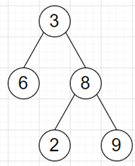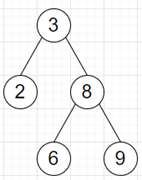# Program to make almost BST to exact BST in python

Suppose we have a binary tree and that is almost a binary search tree. Only two nodes' value are swapped. We have to correct it and return the binary search tree.

So, if the input is likethen the output will beTo solve this, we will follow these steps −

• prev_node := null, min_node := null, max_node := null
• found_one := False
• for each node in the inorder traversal of root, do
• if prev_node is not null, then
• if value of node < value of prev_node, then
• if min_node is null or value of node < value of min_node, then
• min_node := node
• if max_node is null or value of max_node < value of prev_node, then
• max_node := prev_node
• if found_one is true, then
• come out from the loop
• otherwise,
• found_one := True
• prev_node := node
• swap the values of min_node and max_node
• return root

Let us see the following implementation to get better understanding −

## Example

Live Demo

class TreeNode:
def __init__(self, data, left = None, right = None):
self.val = data
self.left = left
self.right = right

def print_tree(root):
if root is not None:
print_tree(root.left)
print(root.val, end = ', ')
print_tree(root.right)

def __iter__(self):
if self.left:
for node in self.left:
yield node
yield self
if self.right:
for node in self.right:
yield node

setattr(TreeNode, "__iter__", __iter__)
class Solution:
def solve(self, root):
prev_node = None
min_node = None
max_node = None
found_one = False
for node in root:
if prev_node:
if node.val < prev_node.val:
if min_node is None or node.val < min_node.val:
min_node = node
if max_node is None or max_node.val < prev_node.val:
max_node = prev_node
if found_one:
break
else:
found_one = True
prev_node = node
min_node.val, max_node.val = max_node.val, min_node.val
return root

ob = Solution()
root = TreeNode(3)
root.left = TreeNode(6)
root.right = TreeNode(8)
root.right.left = TreeNode(2)
root.right.right = TreeNode(9)
print_tree(ob.solve(root))

## Input

root = TreeNode(3)
root.left = TreeNode(6)
root.right = TreeNode(8)
root.right.left = TreeNode(2)
root.right.right = TreeNode(9)

## Output

2, 3, 6, 8, 9,

Updated on: 02-Dec-2020

91 Views